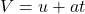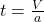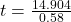## A race car starts from rest on a circular track of radius 378 m. The car’s speed increases at the constant rate of 0.580 m/s2. At the point

Question

A race car starts from rest on a circular track of radius 378 m. The car’s speed increases at the constant rate of 0.580 m/s2. At the point where the magnitudes of the centripetal and tangential accelerations are equal, find the following.

a. The speed of the race car
b. The distance traveled
c. the elapsed time

in progress 0
6 months 2021-07-19T04:55:55+00:00 1 Answers 13 views 0

a)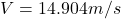b)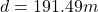c)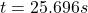Explanation:

From the question we are told that:

Radius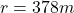Acceleration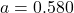a)

Generally the  equation for speed of the car is mathematically given by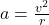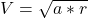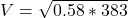b)

Generally the  equation for distance traveled of the car is mathematically given by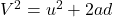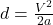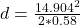c)

Generally the  equation for time of the car is mathematically given by